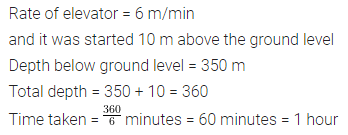# ML Aggarwal Class 7 Solutions for ICSE Maths Chapter 1 Integers Ex 1.4

## ML Aggarwal Class 7 Solutions for ICSE Maths Chapter 1 Integers Ex 1.4

Question 1.
Evaluate the following:
(i) (-36) ÷ (-9)
(ii) 150 ÷ (-25)
(iii) (-270) ÷ 27
(iv) (-59) ÷ 59
(v) 0 ÷ (-17)
(vi) (-784) ÷ (-56)
Solution: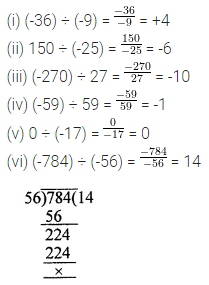Question 2.
Evaluate the following:
(i) 13 ÷ [(-2) + 1]
(ii) (-47) ÷ [(-45) + (-2)]
(iii) [(-6) + 5] ÷ [(-2) + 1]
(iv) [(-48) ÷ (-6)] ÷ (-2)
Solution: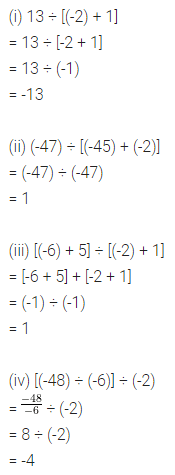Question 3.
Verify that (a ÷ b) ÷ c ≠ a ÷ (b ÷ c) for a = -225, b = 15 and c = -3.
Solution:Question 4.
Verify that a ÷ (b ÷ c) ≠ (a ÷ b) ÷ (a ÷ c) for
(i) a = -10, b = 1 and c = 1
(ii) a = 12, b = 1 and c = -2
Solution:Question 5.
Fill in the blanks to make the following statements true:
(i) 239 ÷ …….. = 1
(ii) (-85) ÷ …….. = -1
(iii) (-213) ÷ ….. = 1
(iv) (-43) ÷ …… = 43
(v) …….. ÷ (-21) = 4
(vi) (-66) ÷ ……. = -3
Solution: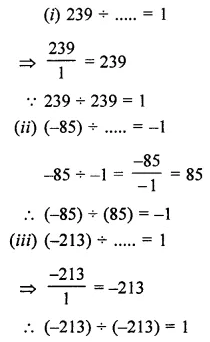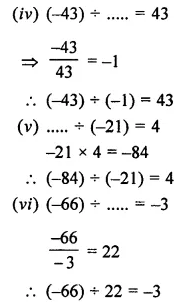Question 6.
Write five pairs of integers (a, b) such that a ÷ b = -3 one such pair is (-6, 2).
Solution:Question 7.
In competition 3 marks are given for every correct answer and (-2) marks are given for every incorrect answer and no marks for not attempting any question.
(i) Sachin scored 24 marks. If he got 14 correct answers, how many questions has he attempted incorrectly?
(ii) Nalini scores (-7) marks in this competition, though she has got 9 correct answers. How many questions she has attempted
incorrectly?
Solution: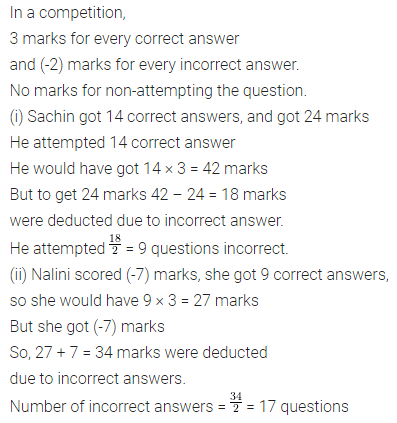Question 8.
An elevator descends into a mine shaft at the rate of 6 m/min. If the descend starts from 10 m above the ground level, how long will it take to reach the shaft 350 m below the ground level?
Solution: